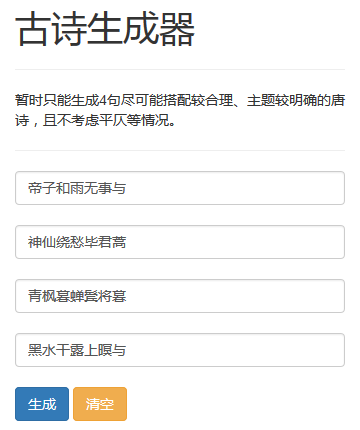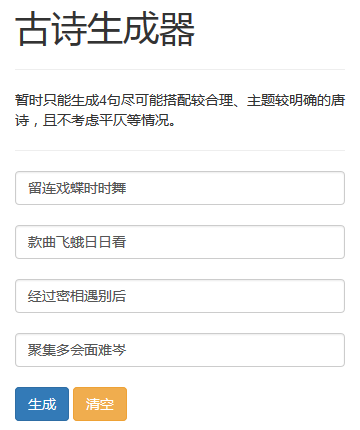## 唐诗生成器

github上repository地址：https://github.com/lijiancheng0614/poem_generator

Python 2.7

jieba

## 运行方法

pip install jieba

python preprocess.py

python get_collocations.py

python get_topic.py

python get_start_words.py

python index.py

## 实现

.data唐诗语料库.txt

.datapoem.txt

.datapoem.txt

.datapoem.txt

.datapoem.txt

### 生成唐诗

maxs.t.∏i=2ncollocations_h_score[a[i−1]][a[i]]+λ∑i=1ntopic_word[topic_id][a[i]]∑i=1nlen(word[a[i]])=la=start_word

f[i][j]=max{f[i−len(word[j])][k]+log_collocations_h_score[k][j]}+λtopic_word[j]

begin{array}{cl}

max & prod_{i = 2}^n collocations_h_score[a[i - 1]][a[i]]

& + lambda_1 prod_{i = 1}^n collocations_v_score[pre_a[i]][a[i]]

& + lambda_2 sum_{i = 1}^n topic_word[topic_id][a[i]]

text{s.t.} & len(word[a[i]]) = len(word[pre_a[i]]),i = 1,cdots,n

end{array}maxs.t.∏i=2ncollocations_h_score[a[i−1]][a[i]]+λ1∏i=1ncollocations_v_score[pre_a[i]][a[i]]+λ2∑i=1ntopic_word[topic_id][a[i]]len(word[a[i]])=len(word[pre_a[i]]),i=1,n

f[i][j]=max{f[i−1][k]+log_collocations_h_score[k][j]+λ1log_collocations_v_score[pre_a[i]][j]}+λ2topic_word[j]

.datacollocations_v

.datacollocations_h

.datawords.txt

.datatopic_words

.datastart_words.txt

### 实现网站## 参考

“结巴”中文分词. https://github.com/fxsjy/jieba ↩TF-IDF. 维基百科. 最后修订于2015年9月27日. https://zh.wikipedia.org/wiki/TF-IDF ↩sklearn.feature_extraction.text.TfidfTransformer. scikit-learn developers. http://scikit-learn.org/stable/modules/generated/sklearn.feature_extraction.text.TfidfTransformer.html ↩Non-negative matrix factorization. Wikipedia. 最后修订于2015年12月1日. https://en.wikipedia.org/wiki/Non-negative_matrix_factorization ↩sklearn.decomposition.NMF. scikit-learn developers. http://scikit-learn.org/stable/modules/generated/sklearn.decomposition.NMF.html ↩He J,Zhou M,Jiang L. Generating chinese classical poems with statistical machine translation models[C]//Twenty-Sixth AAAI Conference on Artificial Intelligence. 2012. ↩

0人推荐

#### 推荐文章

•Python

•Python

•Python

•Python

•Python

•Python

•Python

•Python